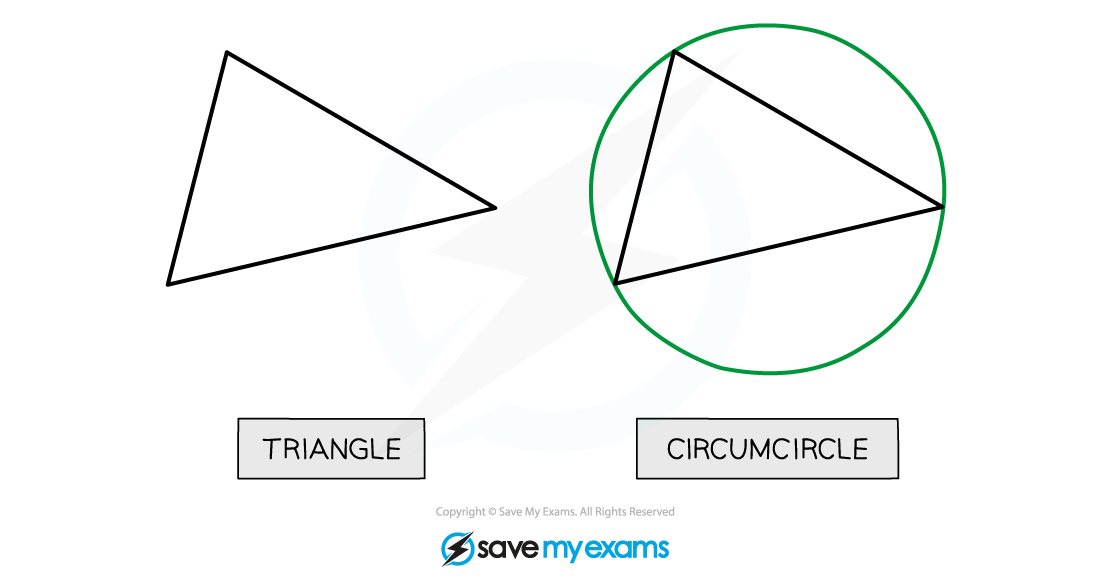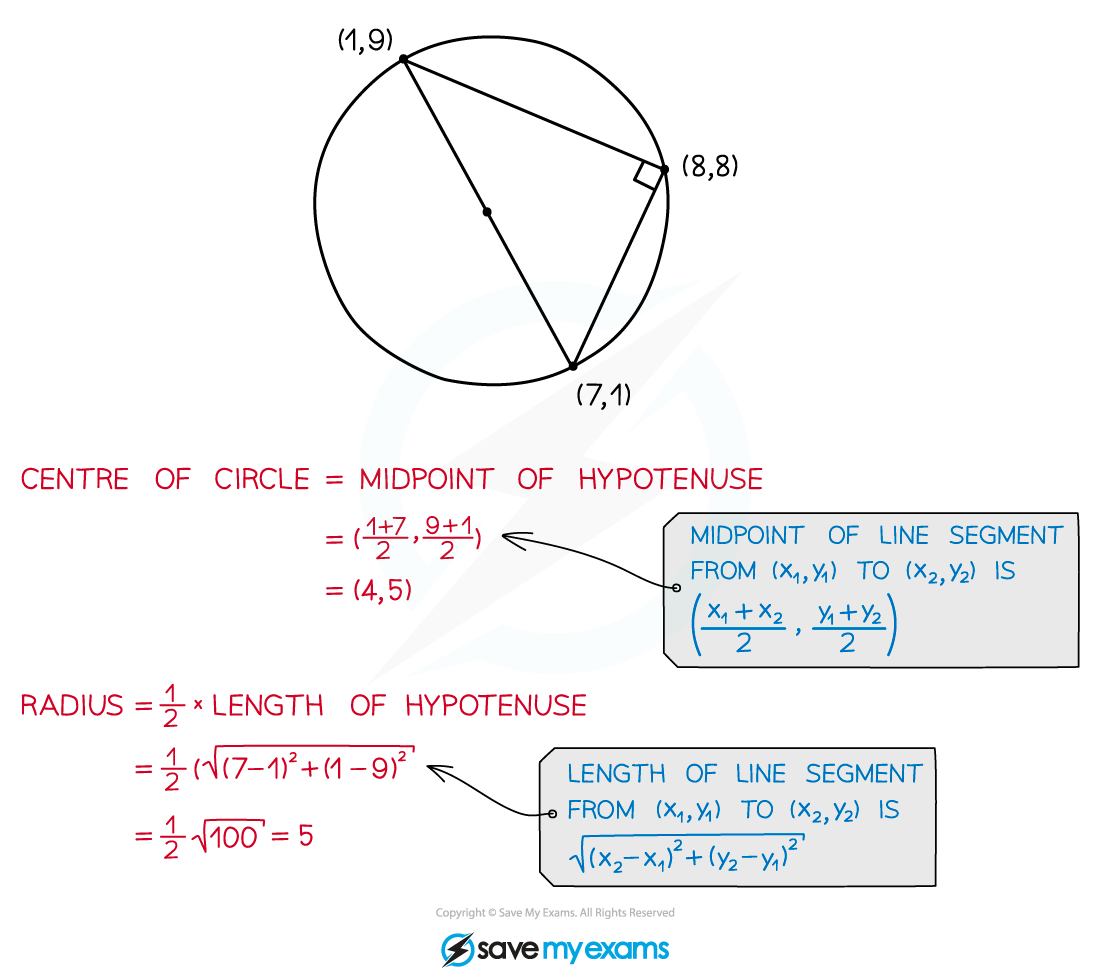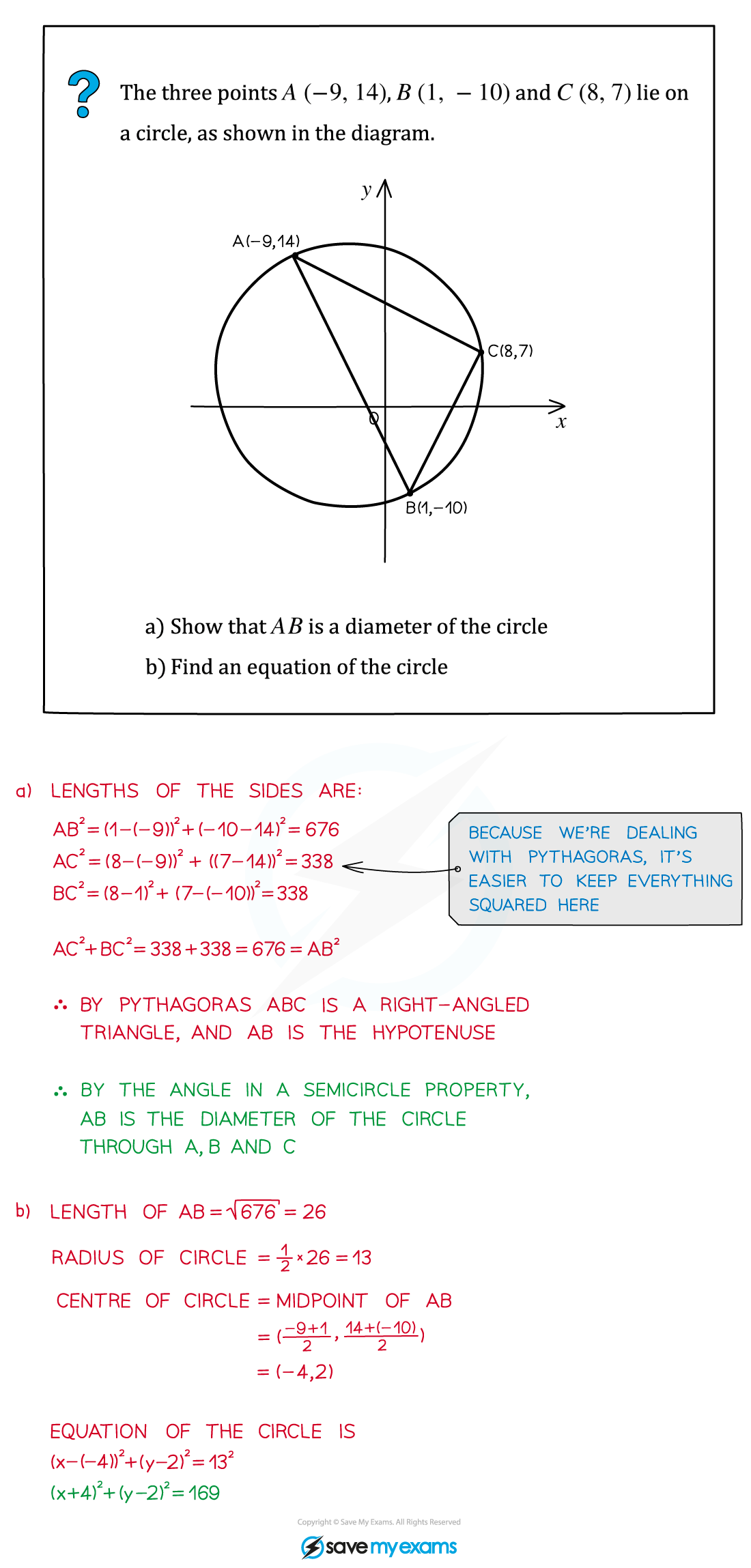# AQA A Level Maths: Pure复习笔记3.2.4 Angle in a Semicircle

### Angle in a Semicircle

#### What is the angle in a semicircle property?

• It is always possible to draw a unique circle through the three vertices of a triangle – this is called the circumcircle of the triangle• The angle in a semicircle property says that If a triangle is right-angled, then its hypotenuse is a diameter of its circumcircle• It also says that any angle at the circumference in a semicircle is a right angle

#### How can I use the angle in a semicircle property to find the equation of a circle?

• Because the hypotenuse of a right-angled triangle is a diameter of the triangle's circumcircle you also know that:
1. the radius of the circumcircle is half the length of the hypotenuse
2. the centre of the circumcircle is the midpoint of the hypotenuse• Once you know the radius and the centre you can write down the equation of the circle#### Exam Tip

• To show that a triangle is right-angled, show that the lengths of its sides satisfy Pythagoras' theorem.

#### Worked Example